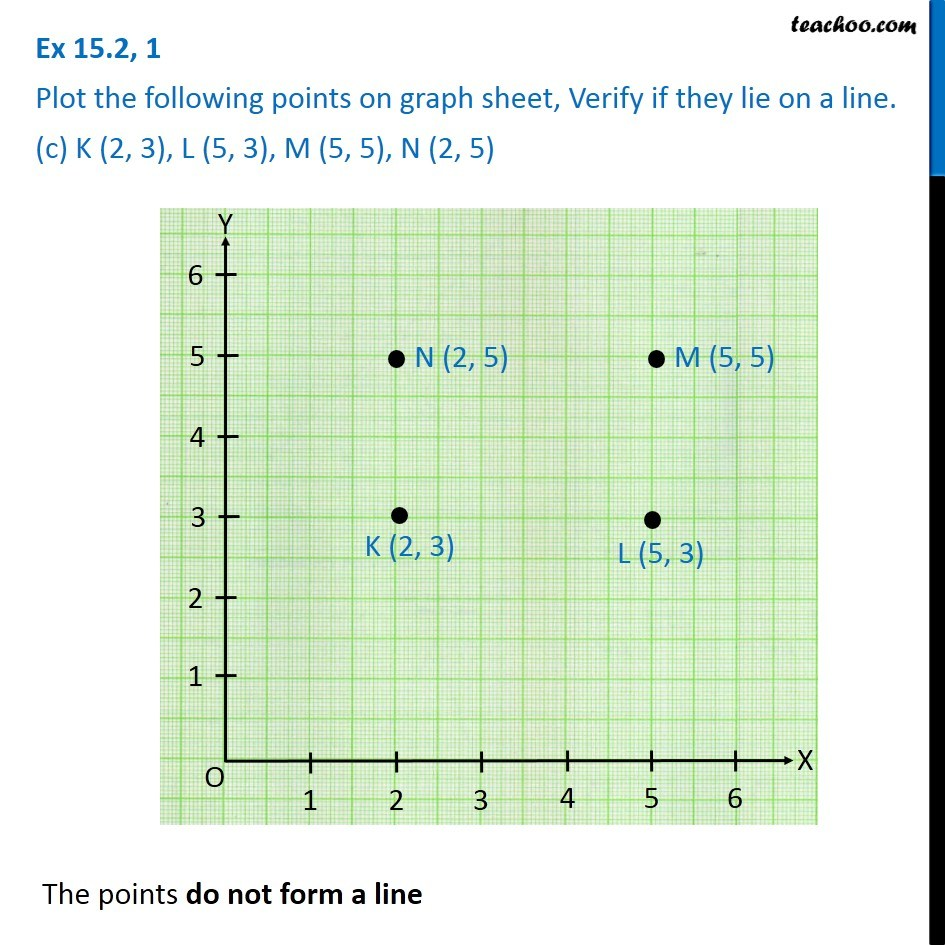1. Chapter 15 Class 8 Introduction to Graphs
2. Serial order wise
3. Ex 15.2

Transcript

Ex 15.2, 1 Plot the following points on graph sheet, Verify if they lie on a line. (c) K (2, 3), L (5, 3), M (5, 5), N (2, 5)The points do not form a line